# For a reaction that follows the general rate law Rate = K[A]°[B][C], what will happen to...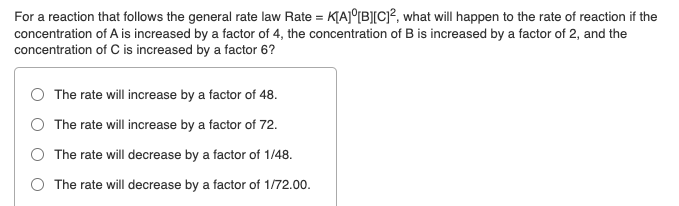For a reaction that follows the general rate law Rate = K[A]°[B][C], what will happen to the rate of reaction if the concentration of A is increased by a factor of 4, the concentration of B is increased by a factor of 2, and the concentration of C is increased by a factor 6? The rate will increase by a factor of 48. The rate will increase by a factor of 72. The rate will decrease by a factor of 1/48. The rate will decrease by a factor of 1/72.00.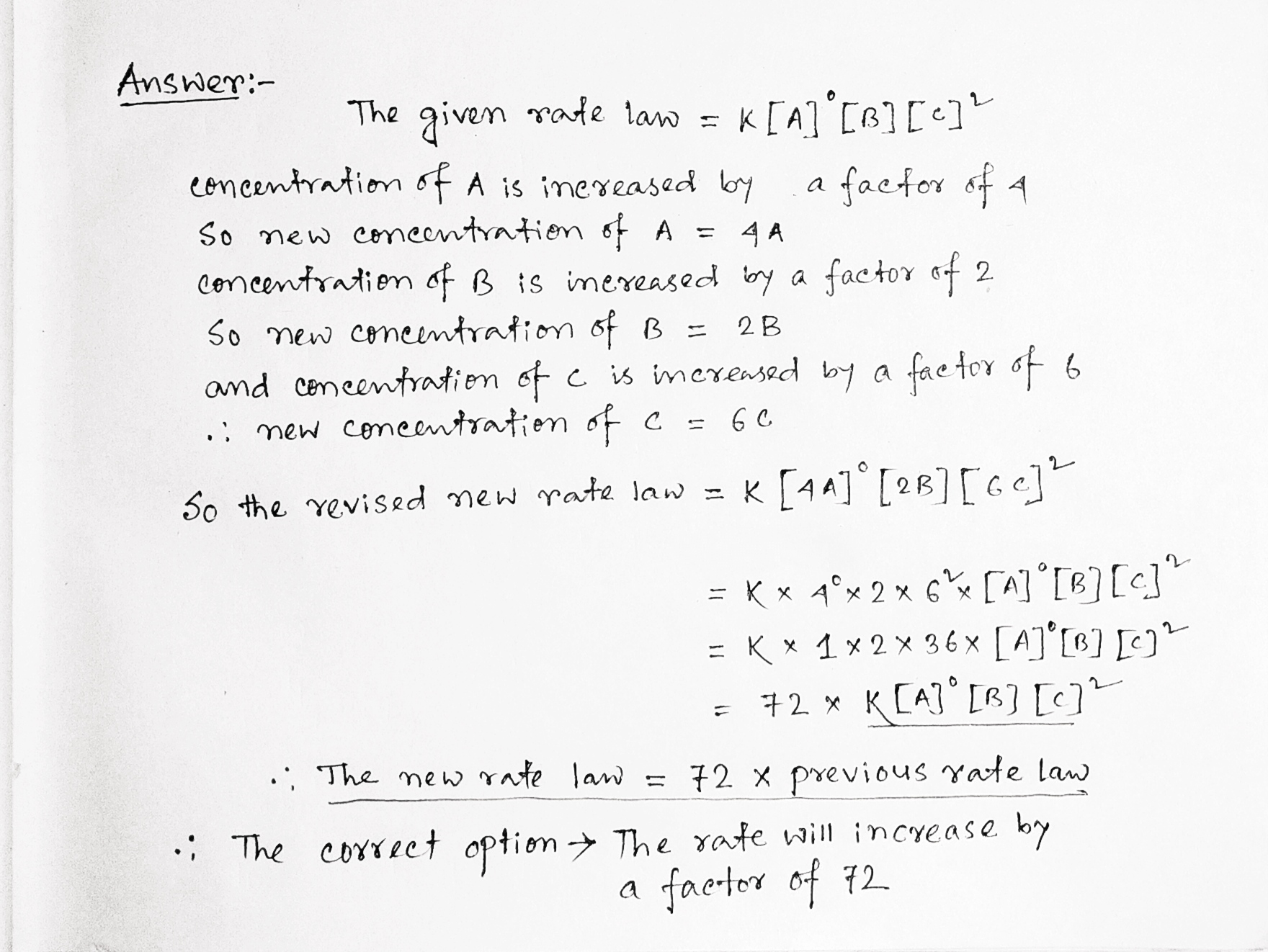##### Add Answer of: For a reaction that follows the general rate law Rate = K[A]°[B][C], what will happen to...
Similar Homework Help Questions
• ### For a reaction that follows the general rate law, rate = k[A]2 [B], what will happen...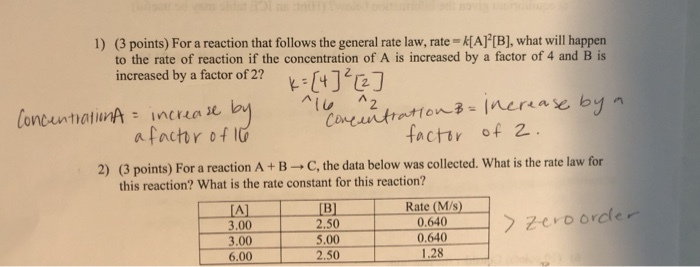For a reaction that follows the general rate law, rate = k[A]2 [B], what will happen to the rate of reaction if the concentration of A is increased by a factor of 4 and B is increased by a factor of 2? 1) (3 points) For a reaction that follows the general rate law, rate = k[A]”[B], what will happen to the rate of reaction if the concentration of A is increased by a factor of 4 and B is...

• ### The reaction A+B → products was found to have the rate law; rate = k[A][B]2. While...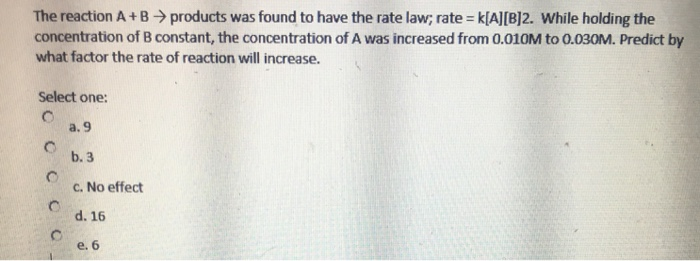The reaction A+B → products was found to have the rate law; rate = k[A][B]2. While holding the concentration of B constant, the concentration of A was increased from 0.010M to 0.030M. Predict by what factor the rate of reaction will increase. Select one: a. 9 b.3 c. No effect d. 16 e. 6 Show transcribed image text

• ### The rate law for the reaction 2A + B - C is found to be rate...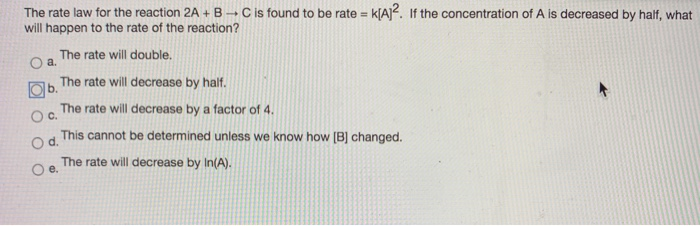The rate law for the reaction 2A + B - C is found to be rate = k[A]2. If the concentration of A is decreased by half, what will happen to the rate of the reaction? O a. The rate will double. The rate will decrease by half. The rate will decrease by a factor of 4. O d. This cannot be determined unless we know how [B] changed. The rate will decrease by In(A). b. C. e. a. Which...

• ### 4. For the reaction A + B + C the rate equation is: rate = k[A]?[B]...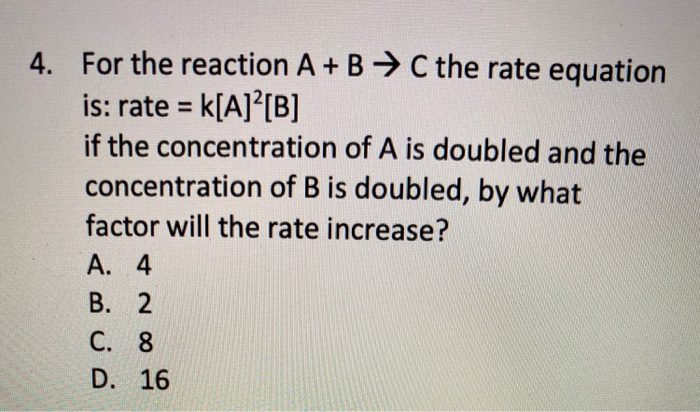4. For the reaction A + B + C the rate equation is: rate = k[A]?[B] if the concentration of A is doubled and the concentration of B is doubled, by what factor will the rate increase? A. 4 B. 2 C. 8 D. 16

• ### 1

For a reaction that follows the general rate law, Rate=k[A][B]^2, what will happen to the rate of reaction if the concentration of B is increased by a factor of 3.00?The rate will...

• ### The rate law for a general reaction involving reactant A is given by the equation rate...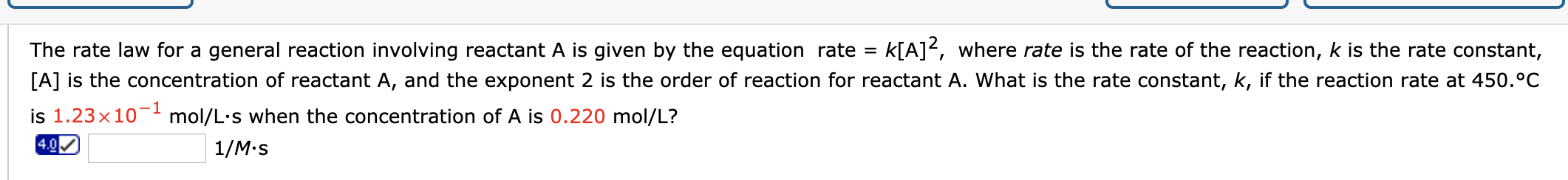The rate law for a general reaction involving reactant A is given by the equation rate = k[A]?, where rate is the rate of the reaction, k is the rate constant, [A] is the concentration of reactant A, and the exponent 2 is the order of reaction for reactant A. What is the rate constant, k, if the reaction rate at 450.°C is 1.23x10-1 mol/L.s when the concentration of A is 0.220 mol/L? 1/Mos When heated to 75°C, 1 mole...

• ### Part A Given the following rate law, how does the rate of reaction change if the...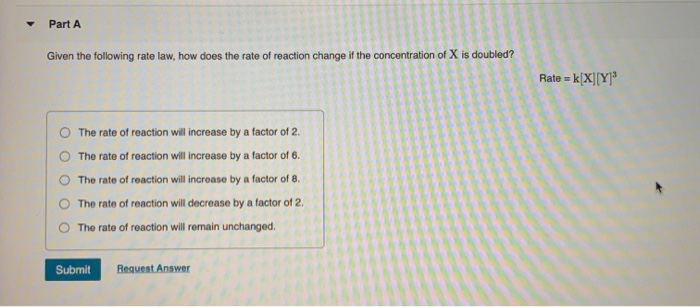Part A Given the following rate law, how does the rate of reaction change if the concentration of X is doubled? Rate = k[X] [Y] The rate of reaction will increase by a factor of 2. The rate of reaction will increase by a factor of 6. The rate of reaction will increase by a factor of 8. The rate of reaction will decrease by a factor of 2. The rate of reaction will remain unchanged. Submit Request Answer Show...

• ### If the rate law for a reaction is rate = k[A]2[B] a. What is the overall...

If the rate law for a reaction is rate = k[A]2[B] a. What is the overall order of the reaction? b. If the concentration of A is doubled and the concentration of B is tripled, how will this affect the rate of the reaction? c. How will doubling the concentration of B while holding A constant affect the value of k (assuming that temperature remains constant)?

• ### 4) A particular irreversible chemical reaction follows the rate law rate = k [A] At a...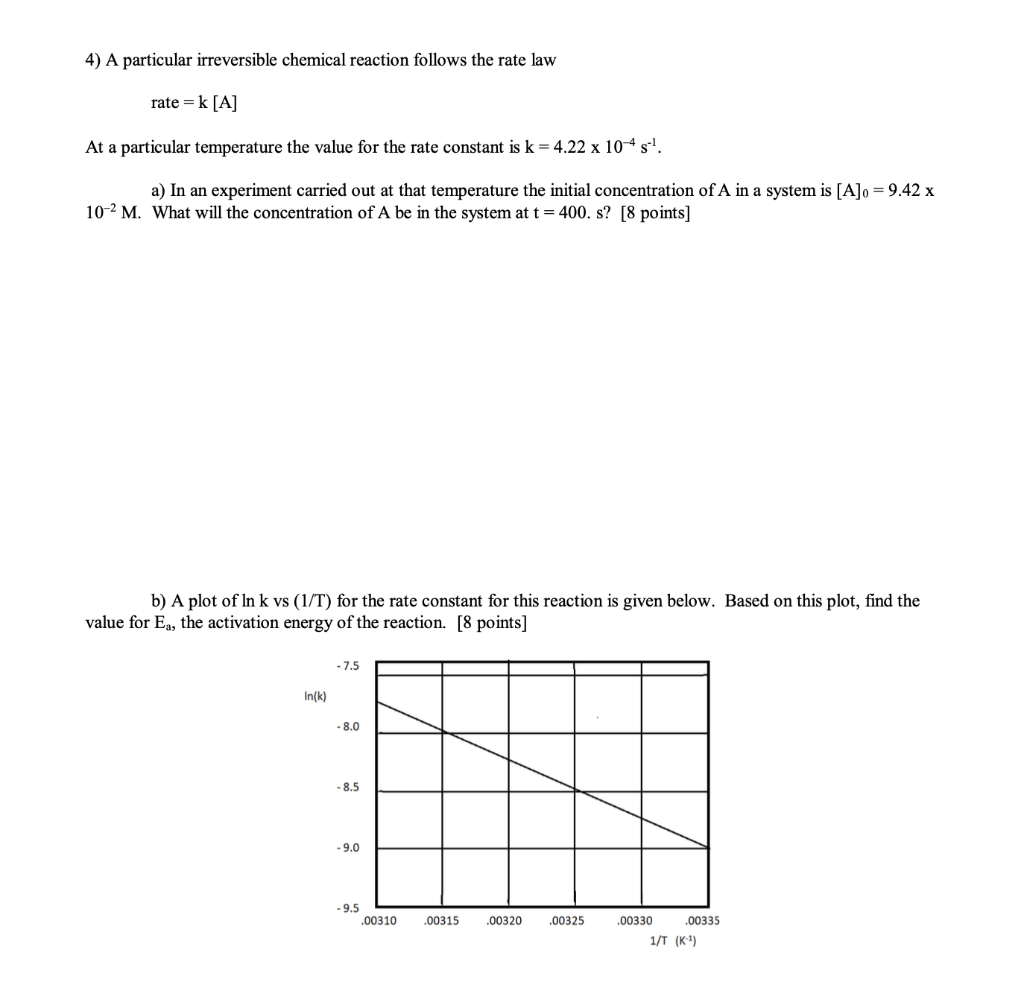4) A particular irreversible chemical reaction follows the rate law rate = k [A] At a particular temperature the value for the rate constant is k = 4.22 x 10-4s!. a) In an experiment carried out at that temperature the initial concentration of A in a system is [A]o = 9.42 x 10-2 M. What will the concentration of A be in the system at t = 400. s? [8 points) b) A plot of In k vs (1/T) for...

• ### 4) A particular irreversible chemical reaction follows the rate law rate = k[A] At a particular...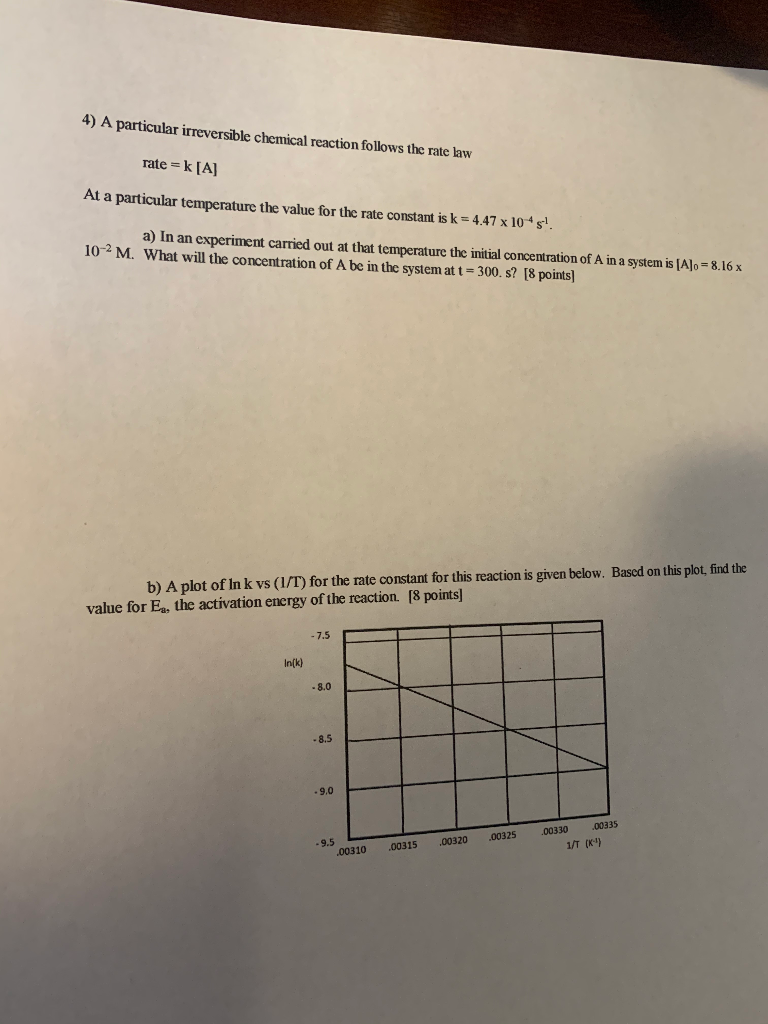4) A particular irreversible chemical reaction follows the rate law rate = k[A] At a particular temperature the value for the rate constant is k = 4.47 x 10*s! a) In an experiment carried out at that temperature the initial concentration of A in a system is [A]o = 8.16 x 10-2 M. What will the concentration of A be in the system at t = 300. s? [8 points) b) A plot of Ink vs (1/T) for the rate...

Free Homework App